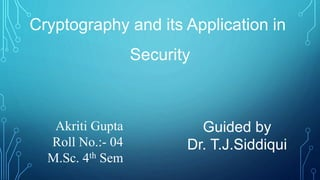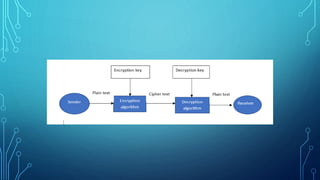Se está descargando tu SlideShare. ×

# Cryptography and its Application in Security

Anuncio
Anuncio
Anuncio
Anuncio
Anuncio
Anuncio
Anuncio
Anuncio
Anuncio
Anuncio
AnuncioCargando en…3
×

1 de 21 Anuncio

# Cryptography and its Application in Security

In the internet & network application, the most challenging aspect is security. With the rapid growth of network and network applications, the security aspect comes first in mind. Cryptography is one of the data security measures. It is invaluable in e-banking, e-trading, and e-commerce. This project involved research and implementation of the three most common existing cryptographic algorithms: RSA, AES, and Blowfish. It was analyzed that given algorithms are the best algorithm for data security.

In the internet & network application, the most challenging aspect is security. With the rapid growth of network and network applications, the security aspect comes first in mind. Cryptography is one of the data security measures. It is invaluable in e-banking, e-trading, and e-commerce. This project involved research and implementation of the three most common existing cryptographic algorithms: RSA, AES, and Blowfish. It was analyzed that given algorithms are the best algorithm for data security.

Anuncio
Anuncio

### Cryptography and its Application in Security

1. 1. Cryptography and its Application in Security Guided by Dr. T.J.Siddiqui Akriti Gupta Roll No.:- 04 M.Sc. 4th Sem
2. 2. INTRODUCTION CRYPTOGRAPHY IS THE SCIENCE OF PROTECTING DATA, WHICH PROVIDES MEANS AND METHODS OF CONVERTING DATA INTO UNREADABLE FORM, SO THAT VALID USER CAN ACCESS INFORMATION AT THE DESTINATION.
3. 3. OBJECTIVE Our main goal in this project is to study and implement existing algorithms of cryptography. We will analyze the various algorithms and then draw conclusion which algorithm is best for data security. Implementation of algorithms of cryptography for securing information includes any type of data-text, image.
4. 4. SERVICES OF INFORMATION SECURITY − • Confidential • Authentication • Integrity • Non-repudiation
5. 5. Types of Cryptography  Symmetric key cryptography Single common key to encrypt and decrypt Faster an Simpler e.g. RSA Algorithm  Asymmetric key cryptography A pair of keys to use to encrypt and decrypt Private and Public key are different.
6. 6. METHDOLOGY  RSA (Rivest-Shamir-Adleman )  AES (Advanced Encryption Standard)  Blowfish
7. 7. In 1977, Rivest, Shamir, and Adelman proposed a scheme using a public key for encrypting messages and a corresponding private key for decryption -this scheme is commonly referred to as RSA. RSA operation RSA algorithm is asymmetric cryptography algorithm. Asymmetric actually means that it works on two different keys i.e. Public Key and Private Key. As the name describes that the Public Key is given to everyone and Private key is kept private. RSA
8. 8. Step 1: Choose two large prime numbers p and q. The product n=p X q is referred to as the modulus and ∅(n). Step 2: Choose an encryption key, e, such that gcd(e,∅(n))= 1. The pair of integers,(e,n) is referred to as the Public key. Step 3: Compute the decryption key, d= 𝑒−1 mod ∅(n). d is also referred to as the private key. Key generation
9. 9. Encryption Let m be the message (or plaintext). We use 𝑚 to denote the length of m. In the naive implementation of RSA, a message is split into multiple blocks, each size b, except possibly for last block. 𝑚 mod b, if different from 0,will be the size of the last block . For each block 𝑚𝑖,calculate the corresponding ciphertext 𝑐𝑖 as 𝑐𝑖=𝑚𝑖 𝑒 mod n (1) Encryption
10. 10. Decryption Given block of ciphertext 𝑐𝑖, the corresponding plaintext is 𝑚𝑖=𝑐𝑖 𝑑 mod n (2) A block of plaintext, 𝑚𝑖 is encrypted as 𝑐𝑖 using (1)
11. 11. AES(advanced encryption standard) Rijndael algorithm supports various blocks sizes-128,192, 256 bits. Likewise, different key sizes may be used -128, 192 , or 256 bits. AES employs 10 rounds for 128-bit keys, and 12 rounds for 192 and 14 rounds for 256 bit keys. It is convenient to visualize a block (and its transformation during and after each round) as a 4 X 4 array of bytes. We refer to this as the state array and denote it as Ƭ.
12. 12. Encryption In the case of 128-bit AES, each round(except for last) employs the following four steps : 1)Byte substitution 2)Row shift 3)Column mixing 4)Round key addition The last round skips the “Column mixing” step. Also, there is a solitary “Round key addition” step just before the first round.
13. 13. Decryption Decryption can proceed in the reverse order -from the 10th round upwards with each step being substituted by its inverse operation. We denote their inverses as 1) InverseByte substitution 2) InverseRow shift 3) InverseColumn mixing 4) InverseRound key addition
14. 14. Blowfish In 1993 an alternative to DES Encryption Technique Blowfish is an encryption technique designed by Bruce Schneier . Block Size: 64-bits Key Size: 32-bits to 448-bits variable size Number of subkeys: 18 [P-array] Number of rounds: 16 Number of subsitution boxes: 4 [each having 512 entries of 32-bits each]
15. 15. Thanks